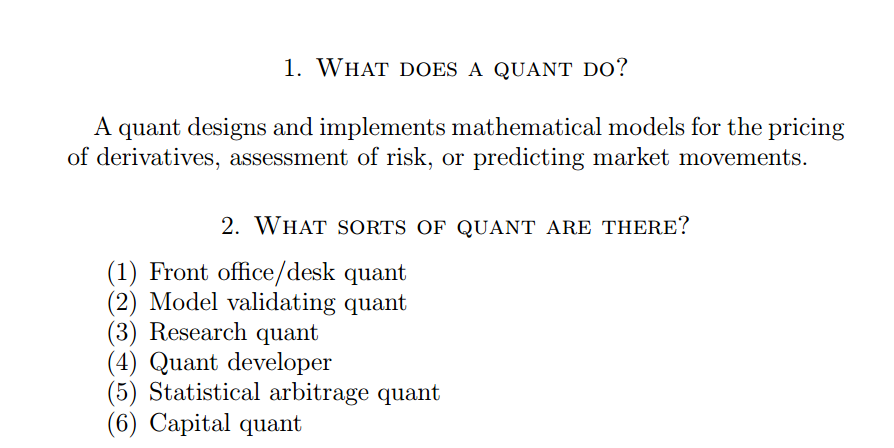## October 18, 2019

### What constitutes Quant Trading and what does not?The operative word here is “mathematical models”.  Of course this can include probabilistic and statistical models as well.

So to sum it up, Quant trading is the application of Mathematics, Statistics and Probability theory in the area of trading.

For example, if you are looking at a simple Moving Average Cross over system, you may want to calculate the Conditional Probability and the Probability Distribution for various outcomes in terms of price. But merely trading a Moving Average Cross over system without having done the statistical work does not qualify as Quant trading.